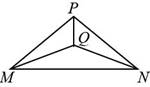Chapter 3.3, Problem 39E### Elementary Geometry for College St...

6th Edition
Daniel C. Alexander + 1 other
ISBN: 9781285195698

#### Solutions

Chapter
Section### Elementary Geometry for College St...

6th Edition
Daniel C. Alexander + 1 other
ISBN: 9781285195698
Textbook Problem
6 views

# In Exercises 37 to 39, complete each proof.Given: Isosceles Δ M N P with vertex PIsosceles Δ M N Q with vertex Q Prove: Δ M Q P ≅ Δ N Q PTo determine

To prove:

ΔMQP is congruent to ΔNQP.

Explanation

Given:

Isosceles ΔMNP with vertex P and isosceles ΔMNQ with vertex Q are given.

Figure (1)

Properties used:

(1) If three sides of one triangle are congruent to three sides of second triangle, the triangles are congruent (SSS).

(2) In an isosceles triangle two sides are congruent.

Approach:

Consider the given isosceles ΔMNP with vertex P.

Since two sides of isosceles triangle are congruent, therefore MP¯NP¯ in ΔMNP and MQ¯NQ¯ in ΔMNQ

### Still sussing out bartleby?

Check out a sample textbook solution.

See a sample solution

#### The Solution to Your Study Problems

Bartleby provides explanations to thousands of textbook problems written by our experts, many with advanced degrees!

Get Started

#### Find more solutions based on key concepts